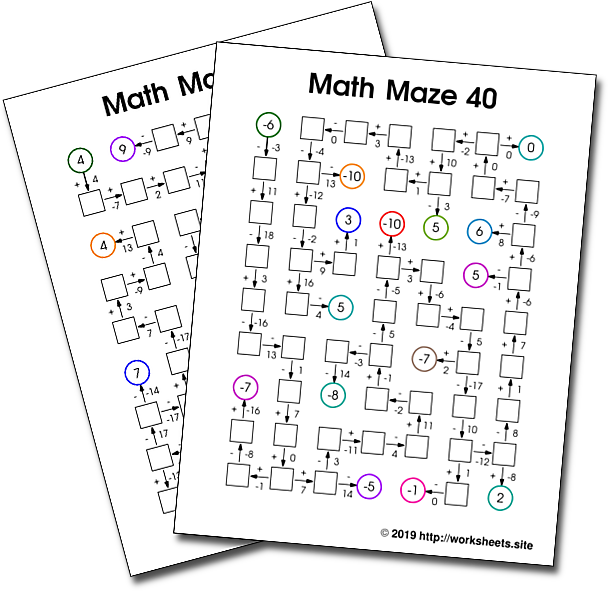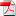# Math Mazes with addition and subtraction and Negative Numbers

Addition and Subtraction Math Mazes with Integers. Free Printable Math Mazes. Fun math activities. Free printable worksheets to practice operations with negative numbers. Fun math worksheets to learn math operations with the whole range of Integer numbers. Pastimes and activities for the middle school math class. 7th Grade Math Activity Sheets. Number Snake No2. Number Snake PDF.## 1. Description

How to master addition and subtraction across zero in a fun way? Try solving these puzzle mazes. Explore the mazes calculating the value of each cell as you move forward. Get all the coins (colored circles). Every time you reach a coin you can compare your result so far with the correct answer and receive immediate feedback.

### 1.1. Importance of immediate feedback

Immediate feedback, and the possibility of correcting errors as soon as we make them, are very effective when we do not have a teacher or tutor present.

Feedback promotes autonomy and self-learning. It is the student who discovers errors and makes correction the moment they arise. Students can work on their own, and at their own pace, making the materials more adaptable to each individual.

Feedback can be a very powerful motivator and promote personal goals to overcome the next task.

In summary, immediate feedback has the potential to help academic performance, promote motivation, self-regulation and self-efficiency, allowing students to narrow the gap between their current performance and their desired performance.

The file has 40 Number Snakes for Addition and Subtraction, one per sheet. Sometimes the student will need to calculate a value by adding, sometimes by subtracting. The numbers range from -20 to 20. Each worksheet requires about 60 calculations.

The booklet is in PDF formatThis is a pencil and paper activity that does not require preparation. Early middle school students only needs the printed sheets and a pencil to use these personal puzzles. The students write their calculations in the boxes as they explore every chamber of the maze.